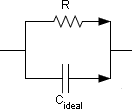Main >> Applications >> Sample problems

# Dielectric losses

capacitor dielectric loss, capacitor loss tangent, nonideal dielectric capacitor, dielectric losses calculation

Simulation of the dielectric losses in the capacitor with known loss tangent.

Problem Type
Plane-parallel problem of AC conduction.

Geometry

d=1 mm, h=10 mm.
The length of capacitor in z direction is l = 10 mm.

Given
Relative permittivity of substrate ε = 2.3.
Loss tangent tgδ = 0.75%.
Voltage U = 220 V,
Frequency f = 100 kHz.

Calculate dielectric losses in capacitor.

Solution
Capacitor with nonideal dielectric can be represented by electric circuit with ideal capacitor C and resistivity R connected in parallel, so that R corresponds to the insulation resistance.
For plane capacitor the capacitance can be calculated as C = εε0·S/d, where S=l·h is a plate surface area.
For plane capacitor the resistance can be calculated as R = (1/γ)·d/SIn the AC conduction problems electric conductivity (γ) of materials should be set. We can derivate the electric conductivity value using the formulae:
tg(δ) = XC/R, where
XC - capacitor reactance, XC = 1 / 2πf·C,
R - capacitor resistance.

After substitution we have:
tg(δ) = 1/ 2πfCR = 1 / 2πf·εε0·S/d·(1/γ)·d/S

Electric conductivity is γ = 2πf·εε0·tg(δ)

Results
Electric conductivity is γ = 2·3.142·100000·8.854e-12·2.3·0.0075 = 0.096 uS/m.Active power dissipated in dielectric is PA = 0.46 mW.

• Video: Dielectric losses
• View simulation report in PDF
• Download simulation files (files may be viewed using any QuickField Edition).
•